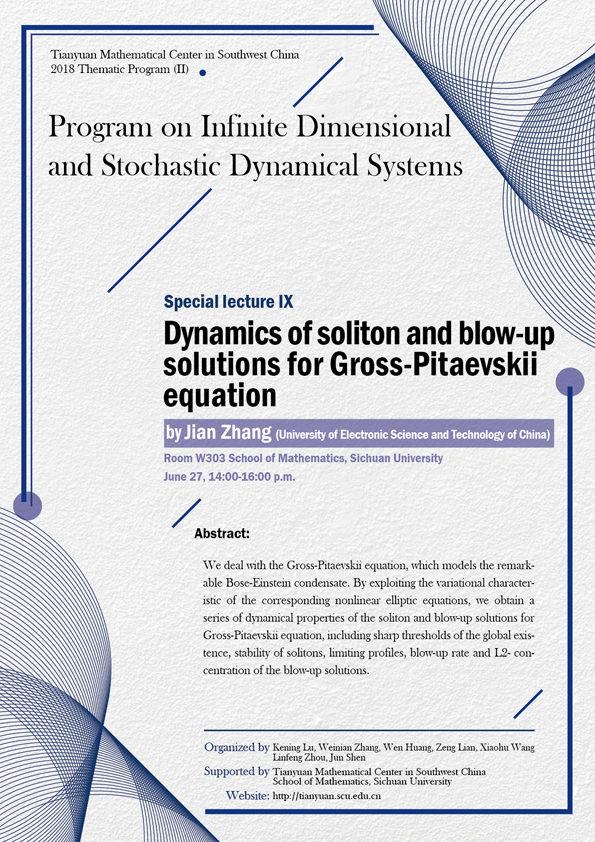2018 Thematic Program (II)

### Dynamics of soliton and blow-up solutions for Gross-Pitaevskii equation

#### W303  School of Mathematics, Sichuan University#### SPEAKER

Jian Zhang (University of Electronic Science and Technology of China)

#### ABSTRACT

We deal with the Gross-Pitaevskii equation, which models the remarkable Bose-Einstein condensate. By exploiting the variational characteristic of the corresponding nonlinear elliptic equations, we obtain a series of dynamical properties of the soliton and blow-up solutions for Gross-Pitaevskii equation, including sharp thresholds of the global existence, stability of solitons, limiting profiles, blow-up rate and L2- concentration of the blow-up solutions.

#### ORGANIZERS

Kening Lu (Sichuan University)

Weinian Zhang (Sichuan University)

Wen Huang (Sichuan University)

Zeng Lian (Sichuan University)

Xiaohu Wang (Sichuan University)

Linfeng Zhou (Sichuan University)

Jun Shen (Sichuan University)

#### SUPPORTED BY

Tianyuan Mathematical Center in Southwest China

School of Mathematics, Sichuan University